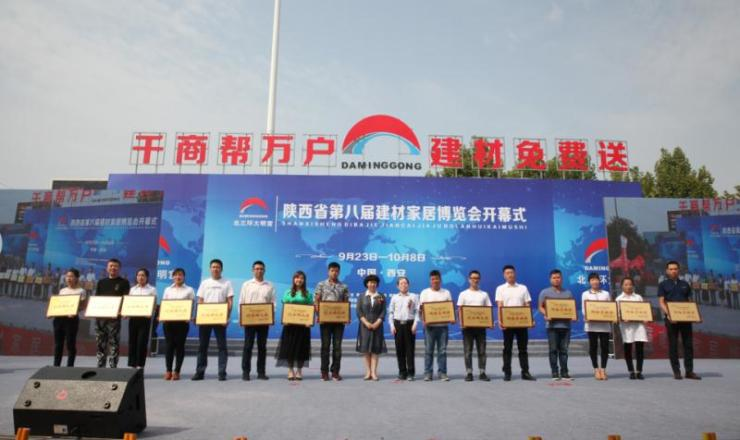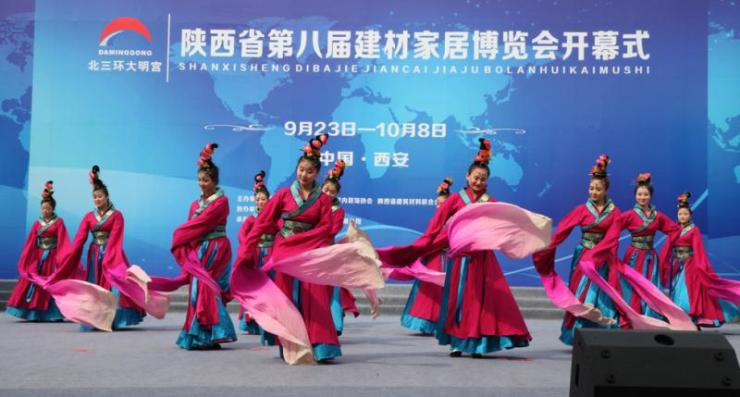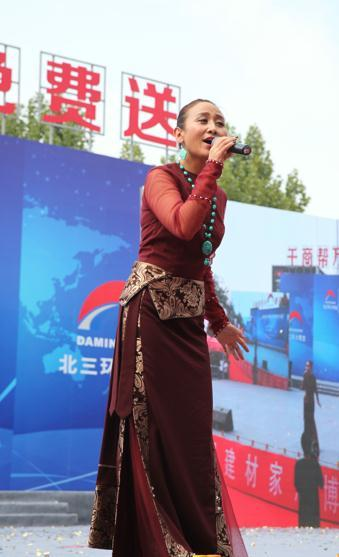|

# 陕西省第八届建材家居博览会9月23日盛大开幕

一年一届的陕西省建材家居博览会于9月23日上午10点，在西安北三环大明宫建材家居批发基地中心广场盛大开幕，全程16天。陕西省建材家居博览会是我省唯一以实体批发基地为会场的大型展会，从2010年至今，已经连续成功举办七届，会期吸纳人流总量超过100万人次，交易总额突破200亿元，为参与活动的商家构建覆盖全省的渠道销售网络，迅速抢占区县级建材市场份额，占尽了先机，占领了财富制高点。

本届的陕西省第八届建材家居博览会由陕西省建材商会、陕西省室内装饰协会、陕西省建筑材料联合会、陕西省家具协会联合主办，西安市装饰业协会鼎力协办，西安大明宫建材家居股份有限公司全力承办。本届博览会以“千商帮万户 建材免费送”为主题，聚焦“八大亮点”，精彩纷呈。亮点一：8大类行业奖项首次揭晓

本届博览会我们将会首次揭晓8大类行业奖项，分别为网络营销奖、安全运营奖、环保贡献奖、慈善扶贫奖、创业孵化奖、服务提升奖、诚信经营奖、管理创新奖，届时在博览会上将会有省、市五大协会的联合授牌、展示。

这份荣誉非同小可，一是授牌的五家协会分别都代表陕西省建材行业发展的最高水平，他们的授牌含金量十足；二是首次揭晓的8大类行业奖项，经过公开、公平、公正的层层选评，网络上更是有10万余人对品牌进行了投票，最终获奖的名单做到了精益求精，其专业性和权威性可想而知；三是参选的建材单位众多，入选品牌多为同类产品中百里挑一者，品质有保证，社会口碑佳；四是观摩人数多，预计过万名业主现场观礼，为品牌加冕。

亮点二：80天3D家装效果图免费出

装修房子考验的是人们的耐心与品味，它是打造一件巨大艺术品的一个过程，而想要一个完美居所，前提必须有一个心仪的装修效果图。此次80天3D家装效果图免费出就是西北首家一站式家装集成服务平台——大明宫家居装修体验馆的专项活动，从8月24日到11月11日，业主可持平面户型图到明宫家居装修体验馆，体验馆将免费向业主设计出各种不同风格的3D家装效果图。同时，从8月24日-9月23日，凡在体验馆下定的业主，将会免费获得由北三环大明宫赠送的花洒一套。除此之外，博览会期间，体验馆更是推出了266元每平方的半包价和666元每平方的全包价。让广大业主省心又省力，安心又踏实。

亮点三、800张电子券线上免费领

这一亮点是北三环大明宫建材家居批发基地联手5000家商户，共同打造的本地建材家居网上采购第一平台——大明宫商城的专项活动，是想通过博览会这个平台，将北三环大明宫网上商城更进一步推广。博览会期间，业主只要登录大明宫商城网，按照程序操作，报名成功后，就能无门槛领取品牌花洒一个。

据了解，目前，大明宫商城已为基地99%以上的商户开通了GPS导航，且在商城上开设网店展示产品的商户有一千多家，同时，商城还经营着北三环大明宫的自营家居。

亮点四、8000品牌花洒免费送

众所周知，北三环大明宫多年来一直在扶贫惠民的道路上一往无前，为保障房低收入群体、城改回迁住户、驻地困难群众,先后斥资6000余万元惠及民生。此次北三环大明宫更进一竿，斥巨资联合基地商户向社会免费送出8000套品牌花洒，有TOTO、九牧、东鹏、惠达、箭牌、中宇、安华、法恩莎等知名品牌，让优质建材惠及万户千家。具体领取方式和注意事项，请关注北三环大明宫微信公众号或拨打029-86482321进行了解。

亮点五、80000件建材半价拿

博览会要做大，做强，做出特色，最重要的是要带动商户参与，要充分发挥商户自己的力量。本届博览会，北三环大明宫发动基地5000家商户集体让利，筹备了声势浩大的半价联盟活动，80000件建材，全部半价拿。

亮点六、北三环大明宫首届大牌超级购

本届博览会，北三环大明宫还有一场重头戏——首届大牌超级购，地点在大明宫家居装修体验馆门前广场。这次的超级购于9月23日上午和博览会同步启动，24日结束，共两天，参与商户27家，品牌28个，邀约业主1000户。届时为所有业主送去多项装修大礼！让广大业主一次看遍北三环大明宫全年特惠产品！一次体验最实惠一元秒杀！一次抢尽各种砍价特卖产品！一次尽享总裁价让利！

亮点七、十大品牌商户惠民专场

博览会期间，北三环大明宫有十个知名品牌将会举办专场特卖会，像兔宝宝专卖会，预计到场业主超过1500人。这样的专卖会在16天里会举办10场，可以说让消费者日日有惊喜，天天有奖拿。

亮点八、著名歌手央金兰泽亲临助兴

“遇上你是我的缘，守望你是我的歌，亲爱的亲爱的亲爱的，我爱你，就像山里的雪莲花”这首被誉为“孤独的牧羊女的天籁之作”——《遇上你是我的缘》是著名歌手央金兰泽的成名曲。9月23日让我们相约北三环大明宫，近距离感受这朵“五彩雪莲花”的清馨芬芳，与众不同。总之，陕西省第八届建材家居博览会期间，北三环大明宫五千多家商户将整装待发，几十万种建材品类直面终端，百万建材价格直击底价，让您真切体会到品质卓越，物超所值。

大格局创造大发展，大平台承载大惠民！

`声明：本文由入驻焦点开放平台的作者撰写，除焦点官方账号外，观点仅代表作者本人，不代表焦点立场错误信息举报电话： 400-099-0099，邮箱：jubao@vip.sohu.com，或点此进行意见反馈，或点此进行举报投诉。`A B C D E F G H J K L M N P Q R S T W X Y Z
A - B - C - D - E
• A
• 鞍山
• 安庆
• 安阳
• 安顺
• 安康
• 澳门
• B
• 北京
• 保定
• 包头
• 巴彦淖尔
• 本溪
• 蚌埠
• 亳州
• 滨州
• 北海
• 百色
• 巴中
• 毕节
• 保山
• 宝鸡
• 白银
• 巴州
• C
• 承德
• 沧州
• 长治
• 赤峰
• 朝阳
• 长春
• 常州
• 滁州
• 池州
• 长沙
• 常德
• 郴州
• 潮州
• 崇左
• 重庆
• 成都
• 楚雄
• 昌都
• 慈溪
• 常熟
• D
• 大同
• 大连
• 丹东
• 大庆
• 东营
• 德州
• 东莞
• 德阳
• 达州
• 大理
• 德宏
• 定西
• 儋州
• 东平
• E
• 鄂尔多斯
• 鄂州
• 恩施
F - G - H - I - J
• F
• 抚顺
• 阜新
• 阜阳
• 福州
• 抚州
• 佛山
• 防城港
• G
• 赣州
• 广州
• 桂林
• 贵港
• 广元
• 广安
• 贵阳
• 固原
• H
• 邯郸
• 衡水
• 呼和浩特
• 呼伦贝尔
• 葫芦岛
• 哈尔滨
• 黑河
• 淮安
• 杭州
• 湖州
• 合肥
• 淮南
• 淮北
• 黄山
• 菏泽
• 鹤壁
• 黄石
• 黄冈
• 衡阳
• 怀化
• 惠州
• 河源
• 贺州
• 河池
• 海口
• 红河
• 汉中
• 海东
• 怀来
• I
• J
• 晋中
• 锦州
• 吉林
• 鸡西
• 佳木斯
• 嘉兴
• 金华
• 景德镇
• 九江
• 吉安
• 济南
• 济宁
• 焦作
• 荆门
• 荆州
• 江门
• 揭阳
• 金昌
• 酒泉
• 嘉峪关
K - L - M - N - P
• K
• 开封
• 昆明
• 昆山
• L
• 廊坊
• 临汾
• 辽阳
• 连云港
• 丽水
• 六安
• 龙岩
• 莱芜
• 临沂
• 聊城
• 洛阳
• 漯河
• 娄底
• 柳州
• 来宾
• 泸州
• 乐山
• 六盘水
• 丽江
• 临沧
• 拉萨
• 林芝
• 兰州
• 陇南
• M
• 牡丹江
• 马鞍山
• 茂名
• 梅州
• 绵阳
• 眉山
• N
• 南京
• 南通
• 宁波
• 南平
• 宁德
• 南昌
• 南阳
• 南宁
• 内江
• 南充
• P
• 盘锦
• 莆田
• 平顶山
• 濮阳
• 攀枝花
• 普洱
• 平凉
Q - R - S - T - W
• Q
• 秦皇岛
• 齐齐哈尔
• 衢州
• 泉州
• 青岛
• 清远
• 钦州
• 黔南
• 曲靖
• 庆阳
• R
• 日照
• 日喀则
• S
• 石家庄
• 沈阳
• 双鸭山
• 绥化
• 上海
• 苏州
• 宿迁
• 绍兴
• 宿州
• 三明
• 上饶
• 三门峡
• 商丘
• 十堰
• 随州
• 邵阳
• 韶关
• 深圳
• 汕头
• 汕尾
• 三亚
• 三沙
• 遂宁
• 山南
• 商洛
• 石嘴山
• T
• 天津
• 唐山
• 太原
• 通辽
• 铁岭
• 泰州
• 台州
• 铜陵
• 泰安
• 铜仁
• 铜川
• 天水
• 天门
• W
• 乌海
• 乌兰察布
• 无锡
• 温州
• 芜湖
• 潍坊
• 威海
• 武汉
• 梧州
• 渭南
• 武威
• 吴忠
• 乌鲁木齐
X - Y - Z
• X
• 邢台
• 徐州
• 宣城
• 厦门
• 新乡
• 许昌
• 信阳
• 襄阳
• 孝感
• 咸宁
• 湘潭
• 湘西
• 西双版纳
• 西安
• 咸阳
• 西宁
• 仙桃
• 西昌
• Y
• 运城
• 营口
• 盐城
• 扬州
• 鹰潭
• 宜春
• 烟台
• 宜昌
• 岳阳
• 益阳
• 永州
• 阳江
• 云浮
• 玉林
• 宜宾
• 雅安
• 玉溪
• 延安
• 榆林
• 银川
• Z
• 张家口
• 镇江
• 舟山
• 漳州
• 淄博
• 枣庄
• 郑州
• 周口
• 驻马店
• 株洲
• 张家界
• 珠海
• 湛江
• 肇庆
• 中山
• 自贡
• 资阳
• 遵义
• 昭通
• 张掖
• 中卫

1室1厅1厨1卫1阳台

1
2
3
4
5

0
1
2

1

1

0
1
2
3报名成功，资料已提交审核A B C D E F G H J K L M N P Q R S T W X Y Z
A - B - C - D - E
• A
• 鞍山
• 安庆
• 安阳
• 安顺
• 安康
• 澳门
• B
• 北京
• 保定
• 包头
• 巴彦淖尔
• 本溪
• 蚌埠
• 亳州
• 滨州
• 北海
• 百色
• 巴中
• 毕节
• 保山
• 宝鸡
• 白银
• 巴州
• C
• 承德
• 沧州
• 长治
• 赤峰
• 朝阳
• 长春
• 常州
• 滁州
• 池州
• 长沙
• 常德
• 郴州
• 潮州
• 崇左
• 重庆
• 成都
• 楚雄
• 昌都
• 慈溪
• 常熟
• D
• 大同
• 大连
• 丹东
• 大庆
• 东营
• 德州
• 东莞
• 德阳
• 达州
• 大理
• 德宏
• 定西
• 儋州
• 东平
• E
• 鄂尔多斯
• 鄂州
• 恩施
F - G - H - I - J
• F
• 抚顺
• 阜新
• 阜阳
• 福州
• 抚州
• 佛山
• 防城港
• G
• 赣州
• 广州
• 桂林
• 贵港
• 广元
• 广安
• 贵阳
• 固原
• H
• 邯郸
• 衡水
• 呼和浩特
• 呼伦贝尔
• 葫芦岛
• 哈尔滨
• 黑河
• 淮安
• 杭州
• 湖州
• 合肥
• 淮南
• 淮北
• 黄山
• 菏泽
• 鹤壁
• 黄石
• 黄冈
• 衡阳
• 怀化
• 惠州
• 河源
• 贺州
• 河池
• 海口
• 红河
• 汉中
• 海东
• 怀来
• I
• J
• 晋中
• 锦州
• 吉林
• 鸡西
• 佳木斯
• 嘉兴
• 金华
• 景德镇
• 九江
• 吉安
• 济南
• 济宁
• 焦作
• 荆门
• 荆州
• 江门
• 揭阳
• 金昌
• 酒泉
• 嘉峪关
K - L - M - N - P
• K
• 开封
• 昆明
• 昆山
• L
• 廊坊
• 临汾
• 辽阳
• 连云港
• 丽水
• 六安
• 龙岩
• 莱芜
• 临沂
• 聊城
• 洛阳
• 漯河
• 娄底
• 柳州
• 来宾
• 泸州
• 乐山
• 六盘水
• 丽江
• 临沧
• 拉萨
• 林芝
• 兰州
• 陇南
• M
• 牡丹江
• 马鞍山
• 茂名
• 梅州
• 绵阳
• 眉山
• N
• 南京
• 南通
• 宁波
• 南平
• 宁德
• 南昌
• 南阳
• 南宁
• 内江
• 南充
• P
• 盘锦
• 莆田
• 平顶山
• 濮阳
• 攀枝花
• 普洱
• 平凉
Q - R - S - T - W
• Q
• 秦皇岛
• 齐齐哈尔
• 衢州
• 泉州
• 青岛
• 清远
• 钦州
• 黔南
• 曲靖
• 庆阳
• R
• 日照
• 日喀则
• S
• 石家庄
• 沈阳
• 双鸭山
• 绥化
• 上海
• 苏州
• 宿迁
• 绍兴
• 宿州
• 三明
• 上饶
• 三门峡
• 商丘
• 十堰
• 随州
• 邵阳
• 韶关
• 深圳
• 汕头
• 汕尾
• 三亚
• 三沙
• 遂宁
• 山南
• 商洛
• 石嘴山
• T
• 天津
• 唐山
• 太原
• 通辽
• 铁岭
• 泰州
• 台州
• 铜陵
• 泰安
• 铜仁
• 铜川
• 天水
• 天门
• W
• 乌海
• 乌兰察布
• 无锡
• 温州
• 芜湖
• 潍坊
• 威海
• 武汉
• 梧州
• 渭南
• 武威
• 吴忠
• 乌鲁木齐
X - Y - Z
• X
• 邢台
• 徐州
• 宣城
• 厦门
• 新乡
• 许昌
• 信阳
• 襄阳
• 孝感
• 咸宁
• 湘潭
• 湘西
• 西双版纳
• 西安
• 咸阳
• 西宁
• 仙桃
• 西昌
• Y
• 运城
• 营口
• 盐城
• 扬州
• 鹰潭
• 宜春
• 烟台
• 宜昌
• 岳阳
• 益阳
• 永州
• 阳江
• 云浮
• 玉林
• 宜宾
• 雅安
• 玉溪
• 延安
• 榆林
• 银川
• Z
• 张家口
• 镇江
• 舟山
• 漳州
• 淄博
• 枣庄
• 郑州
• 周口
• 驻马店
• 株洲
• 张家界
• 珠海
• 湛江
• 肇庆
• 中山
• 自贡
• 资阳
• 遵义
• 昭通
• 张掖
• 中卫• 手机• 分享
• 设计
免费设计
• 计算器
装修计算器
• 入驻
合作入驻
• 联系
联系我们
• 置顶
返回顶部